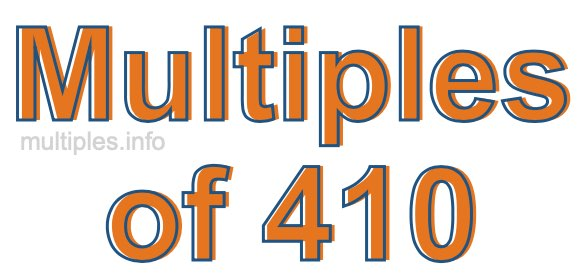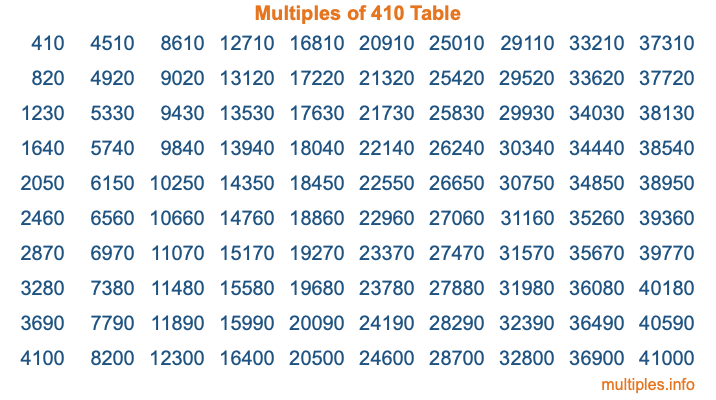Multiples of 410Welcome to the Multiples of 410 page. Here we will first teach you everything you will ever need to know about the multiples of 410, and then give you a study guide summary of everything we taught you to make sure you remember it all. Use this page to look up facts and learn information about the multiples of 410. This page will make you a multiples of four hundred ten expert!

Definition of Multiples of 410
Multiples of 410 are all the numbers that when divided by 410 equal an integer. Each of the multiples of 410 are called a multiple. A multiple of 410 is created by multiplying 410 by an integer.

Therefore, to create a list of multiples of 410, you start with 1 multiplied by 410, then 2 multiplied by 410, then 3 multiplied by 410, and so on for as long as you want. Thus, the list of the first five multiples of 410 is 410, 820, 1230, 1640, and 2050. To see a larger list of multiples of 410, see the printable image of Multiples of 410 further down on this page. We also have a category where you can choose any nth multiple of 410.

Multiples of 410 Checker
The Multiples of 410 Checker below checks to see if any number of your choice is a multiple of 410. In other words, it checks to see if there is any number (integer) that when multiplied by 410 will equal your number. To do that, we divide your number by 410. If the the quotient is an integer, then your number is a multiple of 410.

Is  a multiple of 410?

Least Common Multiple of 410 and ...
A Least Common Multiple (LCM) is the lowest multiple that two or more numbers have in common. This is also called the smallest common multiple or lowest common multiple and is useful to know when you are adding our subtracting fractions. Enter one or more numbers below (410 is already entered) to find the LCM.

Check out our LCM Calculator if you need more details about the Least Common Multiple or if you need the LCM for different numbers for adding and subtraction fractions.

nth Multiple of 410
As we stated above, 410 is the first multiple of 410, 820 is the second multiple of 410, 1230 is the third multiple of 410, and so on. Enter a number below to find the nth multiple of 410.

th multiple of 410

Multiples of 410 vs Factors of 410
410 is a multiple of 410 and a factor of 410, but that is where the similarities end. All postive multiples of 410 are 410 or greater than 410. All positive factors of 410 are 410 or less than 410.

Below is the beginning list of multiples of 410 and the factors of 410 so you can compare:

Multiples of 410: 410, 820, 1230, 1640, 2050, etc.

Factors of 410: 1, 2, 5, 10, 41, 82, 205, 410

As you can see, the multiples of 410 are all the numbers that you can divide by 410 to get a whole number. The factors of 410, on the other hand, are all the whole numbers that you can multiply by another whole number to get 410.

It's also interesting to note that if a number (x) is a factor of 410, then 410 will also be a multiple of that number (x).

Multiples of 410 vs Divisors of 410
The divisors of 410 are all the integers that 410 can be divided by evenly. Below is a list of the divisors of 410.

Divisors of 410: 1, 2, 5, 10, 41, 82, 205, 410

The interesting thing to note here is that if you take any multiple of 410 and divide it by a divisor of 410, you will see that the quotient is an integer.

Multiples of 410 Table
Below is an image of the first 100 multiples of 410 in a table. The table is in chronological order, column by column. The first column has the first ten multiples of 410, the second column has the next ten multiples of 410, and so on.The Multiples of 410 Table is also referred to as the 410 Times Table or Times Table of 410. You are welcome to print out our table for your studies.

Negative Multiples of 410
Although not often discussed or needed in math, it is worth mentioning that you can make a list of negative multiples of 410 by multiplying 410 by -1, then by -2, then by -3, and so on, to get the following list of negative multiples of 410:

-410, -820, -1230, -1640, -2050, etc.

Multiples of 410 Summary
Below is a summary of important Multiples of 410 facts that we have discussed on this page. To retain the knowledge on this page, we recommend that you read through the summary and explain to yourself or a study partner why they hold true.

There are an infinite number of multiples of 410.

A multiple of 410 divided by 410 will equal a whole number.

410 divided by a factor of 410 equals a divisor of 410.

The nth multiple of 410 is n times 410.

The largest factor of 410 is equal to the first positive multiple of 410.

410 is a multiple of every factor of 410.

410 is a multiple of 410.

A multiple of 410 divided by a divisor of 410 equals an integer.

410 divided by a divisor of 410 equals a factor of 410.

Any integer times 410 will equal a multiple of 410.

Multiples of a Number
Here you can get the multiples of another number, all with the same attention to detail as we did for multiples of 410 on this page.

Multiples of
Multiples of 411
Did you find our page about multiples of four hundred ten educational? Do you want more knowledge? Check out the multiples of the next number on our list!

Copyright  |   Privacy Policy  |   Disclaimer  |   Contact